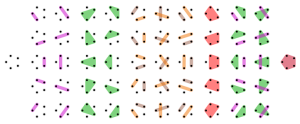This post is devoted to an algorithm due to Aart Johannes Stam for the simulation of the uniform law on the set ${\Pi_n}$ of partitions of ${\{1,\ldots,n\}}$. This law gives the same probability ${1/B_n}$ to every element of ${\Pi_n}$, where ${B_n=\mathrm{Card}(\Pi_n)}$. In combinatorics, ${{(B_n)}_{n\geq1}}$ are the Bell numbers. We have ${B_1=1}$, ${B_2=2}$, and more generally, using the convention ${B_0=1}$,

$B_{n+1}=\sum_{k=0}^n\binom{n}{k}B_k$

(here ${k}$ stands for the number of elements outside the bloc containing ${n+1}$). Now, the formal series ${G(X)=\sum_{n=0}^\infty\frac{B_n}{n!}X^n}$ satisfies to ${G'(X)=\exp(X)G(X)}$, and thus

$G(X)=\exp(\exp(X)-1).$

We recognize the Laplace transform of the Poisson law of unit mean. In particular, the Bell numbers are the moments of this law, which gives the Dobinski formula:

$B_n=\frac{1}{e}\sum_{k=0}^\infty\frac{k^n}{k!}.$

To simulate the uniform law on ${\Pi_n}$, the idea is to associate a random color to each element of ${\{1,\ldots,n\}}$ and to decide that elements of identical color are in the same bloc. More precisely, we first generate a random integer ${K}$ taking the value ${k}$ with probability ${k^n/(k!eB_n)}$ (this is a well defined law thanks to the Dobinski formula above). Next, conditional on ${K}$, we generate i.i.d. random variables ${C_1,\ldots,C_n}$ according to the uniform law on ${\{1,\ldots,K\}}$. Finally, we define the random element ${P}$ of ${\Pi_n}$ obtained by deciding that ${i}$ and ${j}$ are in the same bloc iff ${C_i=C_j}$. It turns out that ${P}$ follows the uniform law on ${\Pi_n}$ since for every ${p\in\Pi_n}$ with say ${b}$ blocs:

$\begin{array}{rcl} \mathbb{P}(P=p) &=&\sum_{k=b}^\infty\mathbb{P}(P=p|K=k)\mathbb{P}(K=k)\\ &=&\sum_{k=b}^\infty\frac{k(k-1)\cdots(k-b+1)}{k^n}\frac{k^n}{k!eB_n}\\ &=&\frac{1}{B_n}. \end{array}$

Exercice. Evaluate the complexity of this algorithm!

References.

Note. I’ve learnt this algorithm while writing an expository text with Yan Doumerc and Florent Malrieu on the chinese restaurant process. It is worth noting that the uniform law on ${\Pi_n}$ does not belong to the Ewens one parameter family of laws on partitions. This is in contrast with the uniform law on permutations, which coincides with the Ewens law of unit parameter.

Note. One should not confuse finite set partitions with integer partitions, which are rather related to Young or Ferrers diagrams.

This site uses Akismet to reduce spam. Learn how your comment data is processed.

Syntax · Style · .